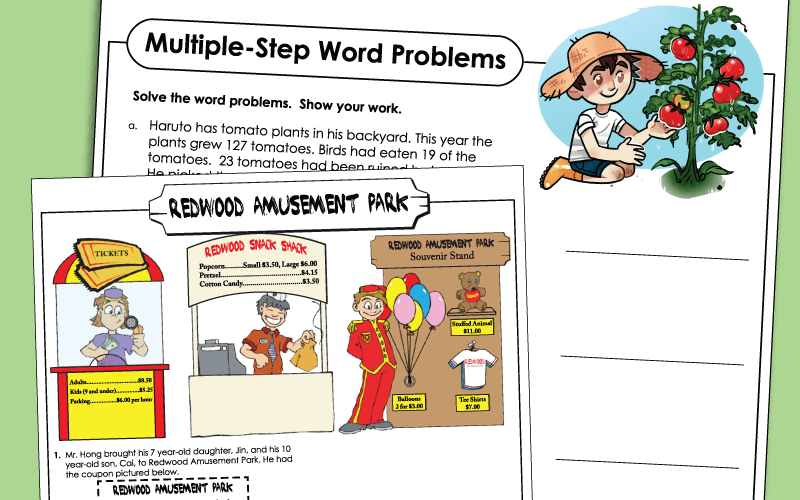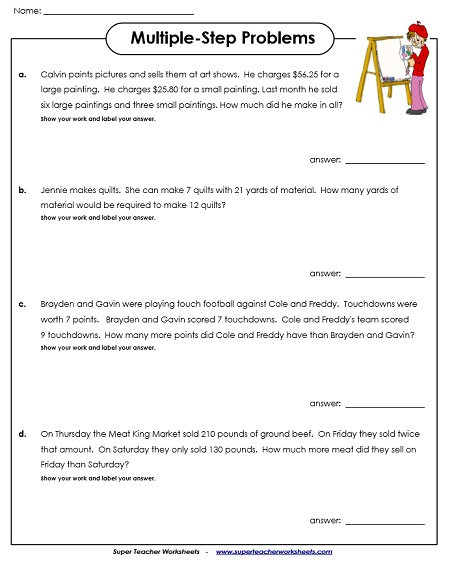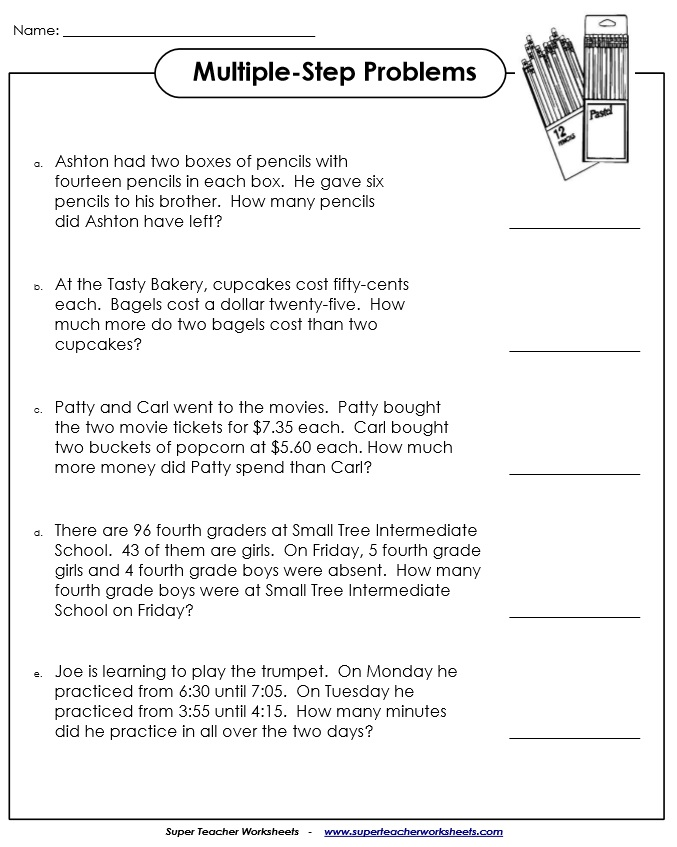# Multiple-Step Word Problems

These multiple-step word problems require students to use reasoning and critical thinking skills to determine how each problem can be solved.Solve each of the multi-step word problems on this page. Problems contain basic numbers of 20 or less. Each problem can be solved without knowledge of multiplication or division.
These multi-step questions can be calculated using only addition and subtraction.
Students will need to add and subtract 2-digit and 3-digit numbers to find the answers to these multi-step word problems.
Challenge your students to solve these tricky multi-step math problems. Each one requires students to add and/or subtract.

These five word problems require students to add and subtract. Includes a money problem and an elapsed time problem.
These word problems have a farming theme. Students use critical thinking to determine which operations are required for solving each problem.
Use addition and subtraction to solve each of these four story problems.
Students solve multi-step problems with an amusement park theme using money. Addition, subtraction, multiplication, and division skills are included.
Solve multi-step problems with a movie theater theme. Skills include add, subtract, multiply, divide, order of operations, and algebraic expressions.
Add to find the total cost of two toys pictured on the shelf. Then subtract across zero to make change.

These four word problems require students to add, subtract, multiply, and divide. Includes a money problem.
Find the answers to the four word problems. Students will need to use reasoning skills to determine whether they should add, subtract, divide, or multiply.
Here are four multiple-step word problems that will require a combination of addition, subtraction, multiplication, or division. Two money questions are included. This file has an answer key.
Practice multi-step problems with these four word problems. Students must use critical thinking to decide whether to add, subtract, multiply, or divide. This worksheet includes one money problem.

Math Word Problems (by Type)

These word problems are sorted by type: addition, subtraction, multiplication, division, fractions and more.

Mixed Skills: Word Problems

These worksheets, sorted by grade level, cover a mix of skills from the curriculum.

Math Worksheets

S.T.W. has thousands of worksheets. Visit the full math index to find them all, sorted by topic.

## Sample Images of Our PrintablesMy Account
Site Information